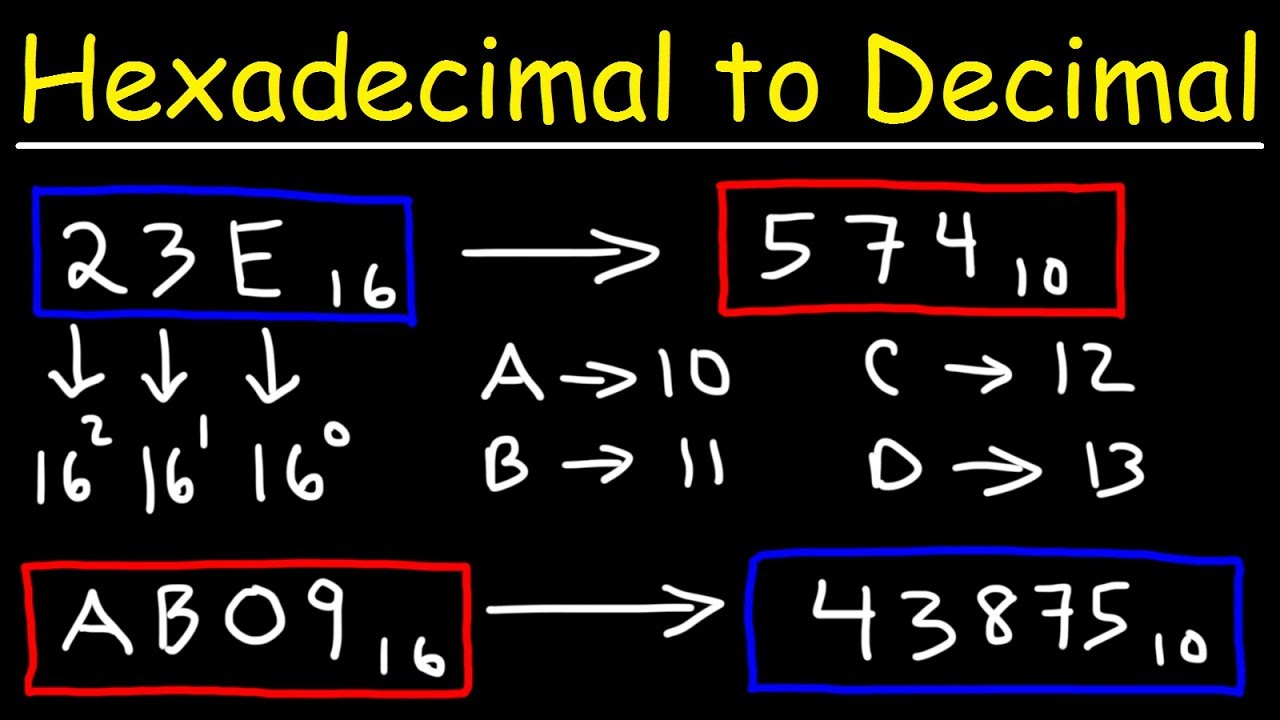# Hex to Decimal Converter

## How to convert from hex to decimal

A regular decimal number is the sum of the digits multiplied with a power of 10.

137 in base 10 is equal to each digit multiplied with its corresponding power of 10:

13710 = 1×102+3×101+7×100 = 100+30+7

Hex numbers are read the same way, but each digit counts the power of 16 instead of the power of 10.

For hex numbers with n digits:

dn-1 … d3 d2 d1 d0

Multiply each digit of the hex number with its corresponding power of 16 and sum:

decimal = dn-1×16n-1 + … + d3×163 + d2×162 + d1×161+d0×160## How to Calculate Hexadecimal to Decimal

Hex is a base 16 number, and decimal is a base 10 number. Therefore, we need to know the decimal equivalent of every hex number digit.

Here are the steps to transform hex to decimal:

• Get the decimal equivalent of hex from the table.
• Multiply every digit with 16 power of digit location.
• (zero-based, 7DE: E location is 0, D location is 1, and the 7 location is 2)
• Sum all the multipliers.

Here is an example:

```7DE is a hex number
7DE = (7 * 162) + (13 * 161) + (14 * 160)
7DE = (7 * 256) + (13 * 16) + (14 * 1)
7DE = 1792 + 208 + 14
7DE = 2014 (in decimal number)```

#### Example #1

3B in base 16 is equal to each digit multiplied with its corresponding 16n:

3B16 = 3×161+11×160 = 48+11 = 5910

#### Example #2

E7A9 in base 16 is equal to each digit multiplied with its corresponding 16n:

E7A916 = 14×163+7×162+10×161+9×160 = 57344+1792+160+9 = 5930510

Example #3

0.8 in base 16:

0.816 = 0×160+8×16-1 = 0+0.5 = 0.510

## Hex to the decimal conversion table

Hex
base 16
Decimal
base 10
Calculation
0 0
1 1
2 2
3 3
4 4
5 5
6 6
7 7
8 8
9 9
A 10
B 11
C 12
D 13
E 14
F 15
10 16 1×161+0×160 = 16
11 17 1×161+1×160 = 17
12 18 1×161+2×160 = 18
13 19 1×161+3×160 = 19
14 20 1×161+4×160 = 20
15 21 1×161+5×160 = 21
16 22 1×161+6×160 = 22
17 23 1×161+7×160 = 23
18 24 1×161+8×160 = 24
19 25 1×161+9×160 = 25
1A 26 1×161+10×160 = 26
1B 27 1×161+11×160 = 27
1C 28 1×161+12×160 = 28
1D 29 1×161+13×160 = 29
1E 30 1×161+14×160 = 30
1F 31 1×161+15×160 = 31
20 32 2×161+0×160 = 32
30 48 3×161+0×160 = 48
40 64 4×161+0×160 = 64
50 80 5×161+0×160 = 80
60 96 6×161+0×160 = 96
70 112 7×161+0×160 = 112
80 128 8×161+0×160 = 128
90 144 9×161+0×160 = 144
A0 160 10×161+0×160 = 160
B0 176 11×161+0×160 = 176
C0 192 12×161+0×160 = 192
D0 208 13×161+0×160 = 208
E0 224 14×161+0×160 = 224
F0 240 15×161+0×160 = 240
100 256 1×162+0×161+0×160 = 256
200 512 2×162+0×161+0×160 = 512
300 768 3×162+0×161+0×160 = 768
400 1024 4×162+0×161+0×160 = 1024

Decimal to hex converter,
Hex to binary converter,
Binary to decimal converter,
Octal to decimal converter,
How to convert hex to decimal,
Hex to ASCII text converter,
Hex to RGB color converter,
Numeral systems,
Number conversion,
10 hex to decimal,
7F hex to decimal,
FF hex to decimal,

Subscribe to Our Youtube Channel SM Funda

Check Full List  Conversion Calculator